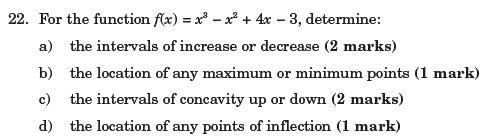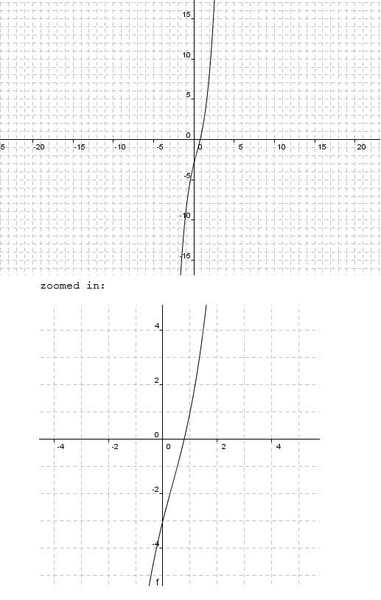# Cubic Functions: Derivatives and Graph Tendencies

## Homework Statement

hey Forum! I had a question here I'm struggling with and was wondering if someone could take a look. its Dealing with calculus, specifically derivatives and behaviors of the graph:

http://i41.tinypic.com/mc6opj.jpgI just started and part a) already has me stumped D:

## The Attempt at a Solution

for intervals of increase or decrease I know we must equate the first derivative to zero.

f(x) = x^3 – x^2 + 4x – 3
f’(x) = 3x^2 – 2x + 4
f’’(x) = 6x – 2

so:
f’(x) = 3x^2 – 2x + 4
0 = 3x^2 – 2x + 4
however I couldn't factor this.. and when I tried to use the quadratic formula.. I got a negative discriminant.
From what I gather this means the derivative has no real roots.. However the actual graph of the original function crosses the x axis just before 1.. I'm confused! how do I calculate this?any advice would be wicked!

Mark44
Mentor

## Homework Statement

hey Forum! I had a question here I'm struggling with and was wondering if someone could take a look. its Dealing with calculus, specifically derivatives and behaviors of the graph:

http://i41.tinypic.com/mc6opj.jpgI just started and part a) already has me stumped D:

## The Attempt at a Solution

for intervals of increase or decrease I know we must equate the first derivative to zero.

f(x) = x^3 – x^2 + 4x – 3
f’(x) = 3x^2 – 2x + 4
f’’(x) = 6x – 2

so:
f’(x) = 3x^2 – 2x + 4
0 = 3x^2 – 2x + 4
however I couldn't factor this.. and when I tried to use the quadratic formula.. I got a negative discriminant.
From what I gather this means the derivative has no real roots.. However the actual graph of the original function crosses the x axis just before 1.. I'm confused! how do I calculate this?any advice would be wicked!
Since f'(x) isn't 0 for any real number x, then there are no points on the cubic's graph with a horizontal tangent. Since the derivative is never zero, it must always be positive or always negative, meaning that the original function is either always increasing or always decreasing.

Thanks! okay that makes a lot of sense actually :D
SO its one or the other, right?

How do I prove whether its constantly increasing or decreasing using derivatives?

cuz normally I would equate the derivative to zero.. take the values that gives me for x..
and consider those 'significant points'.. then I would check the value for the derivative at points after, before, and possibly in between these 'signicant points' to determine where the original function is increasing or decreasing.

but since I have no real values that equate the derivative to zero.. I have no significant points to work with..

and the function crosses the x axis just before x = 1 (according to my graphing calculator)

here is a picture:
http://i41.tinypic.com/bgoaah.jpgbut based on this.. am I correct to say:
there is no minimum or maximum?
We can attest to this simply because the function does is solely ^increasing? is that right?

How do I calculate the point in which the function changes from concave down to concave up, or the point of inflection?
Nvm. I think I got point of inflection.. equate second derivative to zero!
then sub x value into original function! cha ching :D

I know I'm asking alot but this graph really has me grinding my teeth.. thank you so much for helping me through this!

Office_Shredder
Staff Emeritus
Science Advisor
Gold Member
You seem to be confusing f(x)=0 and f'(x)=0. If f'(x) is never zero, then to see whether the function is increasing or decreasing you just evaluate f'(x) at any point (just like you would if you had special critical points, except you only have to check f'(x) at one point instead of a couple)- in this example, f'(0)=4 so the function f(x) is always increasing. The fact that f(x)=0 when x is approximately 1 is no big deal

Aha! Office_Shredder, I see what you mean! you're right, I was jumbling things up there a little bit! Thanks for clearing that up, homie!